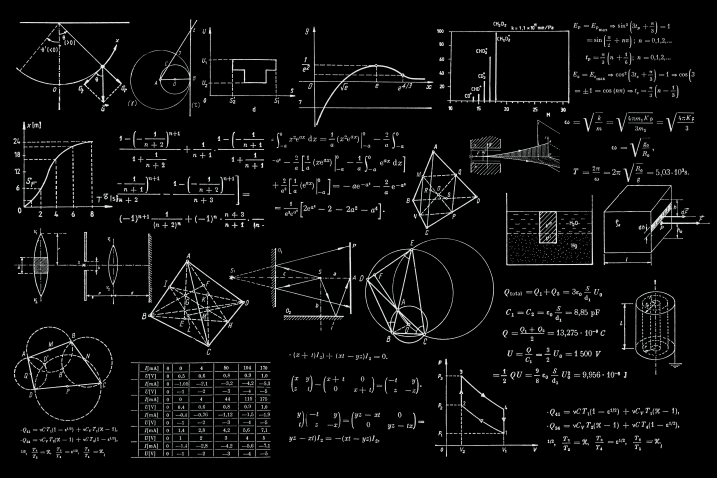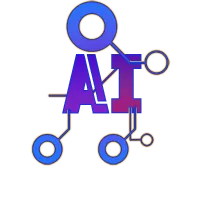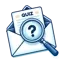Home / Science and Technology / Applied mathematics and number theory

# Applied mathematics and number theory## Information

Applied mathematics and number theory: Applied mathematics and number theory are important branches of mathematics that have significant practical applications in various fields such as engineering, physics, computer science and cryptography among others. Applied mathematics encompasses mathematical methods and techniques used in solving real-world problems. Number theory, on the other hand, is a pure mathematical discipline that studies the properties and behavior of numbers and their relationships with each other. It has numerous applications in cryptography, coding theory and computer science, among other fields. This quiz aims to test your knowledge of basic concepts in applied mathematics and number theory, such as prime numbers, modular arithmetic, number sequences and series, among others.

Categorie: Science and Technology

Keywords: mathematics, applied mathematics, number theory, prime numbers, modular arithmetic, number sequences, coding theory

Total number of questions (advanced mode): 26

Created: 29 mai 2023

Updated: 20 juin 2023Did you know you can also create quizzes with AI? Click on me to learn more!

Attention !! The majority of quizzes on our website are created using AI, so it's possible that some questions or answers may be incorrect. If you notice any, please report the relevant question.### Join the infinite-quiz adventure now!

• Create quizzes with AI
• Earn points
Create my account### Subscribe now

To stay up to date on the latest quizzes, subscribe to our newsletter.

### Our socials